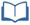# Teacher Resources

## A Good Enough Fit?

On this page you will find lesson objectives, standards, instructional notes and UDL opportunities.

Estimated Time:  60-75 minutes

###Lesson Objectives:

• Students will review linear regression and analyze how well the model fits the data.
• Students will be introduced to residuals and the correlation coefficient and use these tools to determine whether or not a linear model is the best fit for a data set.

Key Common Core State Standards:

S.ID.6 Represent data on two quantitative variables on a scatter plot and describe how the variables are related.

1. Fit a function to the data; use functions fitted to data to solve problems in the context of the data.
2. Informally assess the fit of a function by plotting and analyzing residuals.
3. Fit a linear function for a scatter plot that suggests a linear association.

S.ID.7 Interpret the slope (rate of change) and intercept (constant term) of a linear model in the context of the data.

S.ID.8 Compute (using technology) and interpret the correlation coefficient of a linear fit.

Supporting Common Core State Standards:

F.IF.4 For a function that models a relationship between two quantities, interpret key features of graphs and tables in terms of the quantities, and sketch graphs showing key features given a verbal description of the relationship.

F.LE.1 Distinguish between situations that can be modeled with linear functions and with exponential functions.

Standards for Mathematical Practices:

MP.1 Make sense of problems and persevere in solving them.
MP.2 Reason abstractly and quantitatively.
MP.3 Construct viable arguments and critique the reasoning of others.
MP.4 Model with mathematics.
MP.5 Use appropriate tools strategically.
MP.6 Attend to precision.

## Instructional Notes:

In this lesson, students will be provided with a link to a Shodor Applet.  Students also have the option to use a graphing calculator to graph scatter plots, calculate the linear regression equation, display the residual plot, and calculate the correlation coefficient.

The first scenario allows students to analyze how well a linear model fits the given data.  For this scenario, no model truly fits the data well, but students would initially anticipate that of the functions they know, a linear model would be the best choice.  This scenario provides an entry point into analysis tools, such as correlation coefficient and residuals, to determine the strength of the model selected.  In this case, students will learn that the linear model is not strongly correlated to the data and does not give accurate predictions of future winning times.

Students then apply their understanding of correlation coefficient and residuals to analyze the fit of another data set.  By the end of this lesson, students should begin to analyze each function type (linear, quadratic and exponential) to consider when each model may be appropriate.

As an optional extension or in-class activity, have students collect cooling curve data for other heated beverages and compare.  Use this opportunity to practice calculating an exponential regression equation and calculating residuals to analyze the fit of the model.

> Go to A Good Enough Fit? lesson

## Sample Responses

For sample responses to the Algebra II Journal questions, visit the Algebra II Journal in the Teacher Resources. Students may require additional graph paper for some activities.

## A Good Enough Fit? - Page 5

If students are using a graphing calculator, the calculators will need to have “diagnostic” on.  Calculator instructions are available in the Calculator Resources in the Student Resources section.

## A Good Enough Fit? - Page 12

For sample responses to the Algebra II Journal questions, visit the Algebra II Journalin the Teacher Resources.  Before students begin Journal Reflection 3, remind them that when describing residual values, "small" will be relative to the data set.  Ideally, the individual residual values should be as close to 0 as possible.

> Go to lesson, page 12

## UDL Opportunities:

Checkpoint 2.1 Clarify vocabulary and symbols
Checkpoint 2.3 Support decoding of text, mathematical notation, and symbols
The Student Resources section includes key vocabulary clarifications that will support the decoding of text and mathematical language.  It may be helpful to introduce some of the key terms prior to the start of this lesson.  Students will also need to be comfortable using a graphing calculator.  A quick reference guide will also be available in the Calculator Resources in the Student Resources section.

Checkpoint 3.1:  Activate or supply background knowledge
Prior to this lesson, assess the background knowledge of students informally or formally to determine readiness for this online module.  Students will need to understand key features and behaviors of linear, quadratic and simple exponential functions in order to accurately select a model to represent a given data set.

Checkpoint 3.2:  Highlight patterns, critical features, big ideas, and relationships
By the end of this lesson, students should begin to identify critical features of each function type (linear, quadratic and exponential) as strategies to identify which model is best for a given data set.  Encourage student discussion about critical features and relationships between function families.

Checkpoint 4.1:  Vary the methods for response and navigation
Checkpoint 5:  Provide options for expression and communication
Students have options to use an online applet and/or graphing calculator to analyze the data sets.  The end of lesson reflection may be submitted to the teacher.  Alternatives may include having students or groups present their findings to the class or having students do a gallery walk of final models for scenario 2.

Checkpoint 7.2:  Optimize relevance, value, and authenticity
The scenarios in this lesson are based on real-world data in order to optimize relevance and authenticity.  The optional in-class extension offers students an opportunity to collect and analyze real-world data.